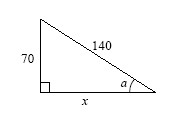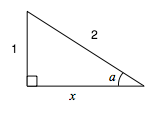### Home > A2C > Chapter 3 > Lesson 3.2.3 > Problem3-126

3-126.

Find $x$and $m∠A$ in the triangle at right.Try making a similar triangle with side lengths divided by the greatest common factor. (70)Remember the side lengths of a 30-60 right triangle.

Or you could use the fact that when you know the sine, your calculator will give you the angle.

m∠A = 30°